Mathematical and Physical Journal
for High Schools
Issued by the MATFUND Foundation
 Already signed up? New to KöMaL?# Exercises and problems in InformaticsOctober 2002

I. 31. In ancient Egypt rational numbers between 0 and 1 were represented by a sum of unit fractions $\displaystyle \frac{1}{x_1}+\frac{1}{x_2}+\ldots+\frac{1}{x_k}$ with xi's being different positive integers. Examples: $\displaystyle \frac{2}{5}=\frac{1}{3}+\frac{1}{15}$,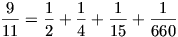, $\displaystyle \frac{19}{30}=\frac{1}{2}+\frac{1}{8}+\frac{1}{120}$.

Your program (I31.pas, ...) should convert a given rational number $\displaystyle \frac{M}{N}$ (1$\displaystyle \le$M<N, 2$\displaystyle \le$N $\displaystyle \le$30) to unit fraction form. (10 points)

I. 32. A bipolygon is obtained by merging the edges of two regular polygons, that is by alternately drawing their edges. (In the figure we merged a square and a triangle by drawing 3 edges of each. We may draw 4 edges as well by connecting the vector U3 to V2 and V0 to U3.)

A multipolygon is produced similarly using more than 2 regular polygons.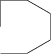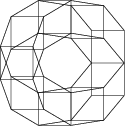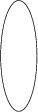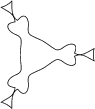DB=2, N=3 H=50, SZ=90 H=50, SZ=120 DB=2, N=40 H=50, SZ=90 H=50, SZ=-40 DB=2, N=360 H=0.5, SZ=1 H=1, SZ=-1 DB=4, N=360 H=1, SZ=1 H=1, SZ=-8 H=1, SZ=16 H=1, SZ=-2

Write your program (I33.pas, ...) which reads the number of polygons to be merged (1$\displaystyle \le$DB $\displaystyle \le$100), the number of edges to be drawn of each polygon (1$\displaystyle \le$N $\displaystyle \le$360), the lengths of the edges and the exterior angles of the polygons (1$\displaystyle \le$H(i)$\displaystyle \le$100 and -120$\displaystyle \le$S(i)120, respectively), then draws the corresponding multipolygon.

See an example on the figure. (10 points)

I. 33. Alice and Bob are playing the following game. First they list N (1$\displaystyle \le$N $\displaystyle \le$100) natural numbers, from which they both choose M (1$\displaystyle \le$M $\displaystyle \le$N) ones. They proceed regularly: Alice selects every Ath number (1$\displaystyle \le$A $\displaystyle \le$N) and Bob selects every Bth one (1$\displaystyle \le$B $\displaystyle \le$N). If the list ends, they continue the selection from the beginning, that is the (N+1)th number will be the first element of the list, the (N+2)th will be the second one, and so on. The winner is the player whose numbers have the greater sum.

Prepare your sheet (I34.xls) which, if N, M, A, B are given, decides the winner. The label of the winner (A or B) should be displayed in red, while that of the loser in blue. If the game ends in a draw, both letters should be black.

See an example in the table.

 N= 10 11 12 13 14 15 16 17 18 19 20 M= 6 A: 3 14 17 20 13 16 19 Sum of A: 99 B: 4 15 19 13 17 11 15 Sum of B: 90

(10 points)